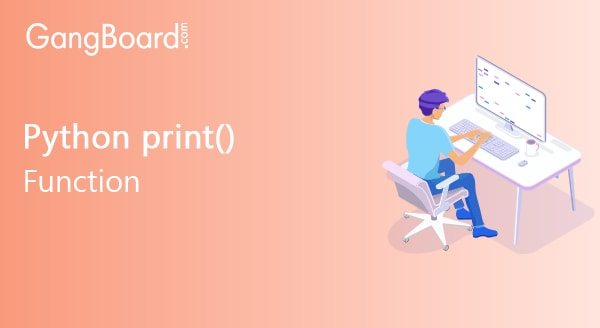# Print() Function in Python

January 14th, 2020

## Print() Function in Python

The print() function is the simplest and easiest way to create output on the screen or console window. As a naive user, one might think this function is useless and not really necessary but in the long run, you will come to realize that this is one of the most useful and effective functions for producing output.
A developer who is set out to debug his program at the point where errors keep occurring can use the print() function to see at which place his program is going astray. Even in other cases, at times when you want to find out when and where your program is behaving incorrectly, the print() function can e effectively used.
Let us first see the very basic implementation of print() function –

### Sample Code :

 ```m = 10000 n = Python print (‘This is’, m, n)```

### Output:

 `This is 10000 Python`

This is a very simple command where the entire logic is very straight forward. The print() function prints all the content specified in the order.

Now let us see how the ‘+’operator is used for the same case to concatenate two different parts of the content.

### Sample code:

 ```x = ‘2020’y = ‘New year’ print ( x+y )```

### Sample Output:

 `2020 New year`

The other syntax used for the print() function is as follows:

 `print ( object(x), separator = sep, end = e, file = f, flush = fl)`

Now let us see in detail what each parameter stands for.

• object(x) – This parameter is used to indicate an object which will be changed to a string format.
• separator = sep – This entire parameter is purely optional. It is meant for the purpose of separating the objects when multiple ones are present.
• end = end – This parameter is again optional. It is present to specify what value should appear at the last end.
• file = f – This parameter is also optional. It is an object with a write method.
• flush = f – This is also an optional parameter.         It is basically a Boolean value that is meant to indicate the option ‘flushed’ if it is set to true and ‘buffered’ if it is set to false.

## Procedure to print blank lines in Python

There can be cases where you have to print blank lines as a part of the program and in such cases Python allows you to use its features to get work done easier. Consider the following example code

 ```print (“Hello! We are Besant Technologies.”) print (“\n\n\n”) print (“End. Thanks for visiting us”)```

Or there is also another way of writing the same code to achieve the same result of printing three blank lines.

 ```print (“Hello! We are Besant Technologies.”) print ( 3 * “\n”) print (“End. Thanks for visiting us”)```

The output for the above pieces of code will look like,

 `Hello! We are Besant Technologies. End. Thanks for visiting us`

As you can clearly see between there are three empty lines appearing between the first line of text and the last line of text.

### Command – print end :

In Python, automatically the print() method ends every time with a fresh new line. It is mainly because; this method or function comes with the ‘end’ parameter. And this parameter has the value ‘\n’ by default. One can include this parameter to end any print statement. Consider the following piece of sample code.

 ```print (“Good Morning!”, end = ‘ ‘) print (“This is Besant Technology!”, end = ‘!‘)```

The output for the following sample code will look like:

 `Good Morning! This is Besant Technology!!`

As you can very well see, in this piece of code, in the first line, the text mentioned within the print command is printed promptly. Owing to the end = ‘ ‘ command present at the end, space occurs. And then following that, the second line of text within the print command gets printed successfully. As we have specified that end = ‘!’ within brackets, it indicates that the specifically an exclamation mark should appear at the end of the printed text. So accordingly the last exclamation gets printed.

### Use of parameter – flush :

The parameter called ‘flush’ which is available in Python gives the user the option to choose if one wants buffered/unbuffered output. By default, this parameter has the value ‘False’ which indicates the presence of buffered output. If the user decides to set this value to ‘True’, then unbuffered output is obtained. But one disadvantage is the fact that the latter process is way slower when compared to the earlier one.  Consider the following example to understand the working of the ‘flush’ parameter in a better way.

Sample code:

 ```import timex = open ( ‘python.txt’ , ’r’ ) m = x.read() n = time.time() print (m, flush = True) o = time.time() print ( o+n)```